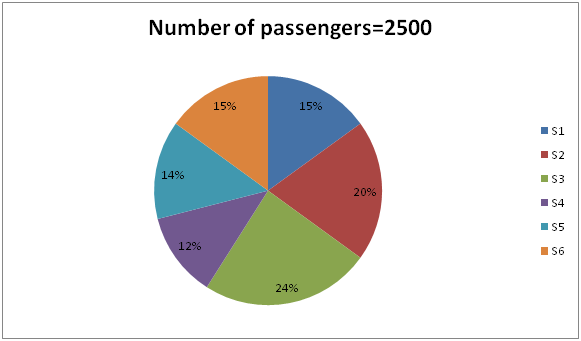# IBPS PO Prelims Quantitative Aptitude Questions 2019 (Day-13)

Dear Aspirants, Our IBPS Guide team is providing new series of Quantitative Aptitude Questions for IBPS PO Prelims 2019 so the aspirants can practice it on a daily basis. These questions are framed by our skilled experts after understanding your needs thoroughly. Aspirants can practice these new series questions daily to familiarize with the exact exam pattern and make your preparation effective.

Check here for IBPS PO Prelims Mock Test 2019

##### Check here for IBPS PO  Mock Test 2019
[WpProQuiz 7122]

Direction (1 – 5): Find the wrong term in the following number series.

1) 38, 137, 217, 281, 328, 363, 387

a) 281

b) 328

c) 137

d) 387

e) None of these

2) 11, 26, 55, 116, 236, 476, 956

a) 116

b) 55

c) 476

d) 236

e) None of these

3) 23, 42, 80, 156, 306, 612, 1220

a) 42

b) 306

c) 1220

c) 156

e) None of these

4) 28, 13, 28, 98, 490, 3185, 25480

a) 13

b) 98

c) 490

d) 3185

e) None of these

5) 13, 76, 137, 195, 253, 308, 361

a) 253

b) 361

c) 195

d) 137

e) None of these

Direction (6 – 10): Study the following information carefully and answer the given questions:

The given pie graph shows the number of passengers in 6 different coaches of train.6) The number of passengers in coaches S1 and S2 together is approximately what percent of the number of passengers in coaches S6 and S3 together?

a) 90%

b) 85%

c) 80%

d) 82%

e) None of these

7) If in coach S4, 25% of the passengers are female and 20% is children, then what is the number of male passengers in coach S4?

a) 135

b) 145

c) 155

d) 165

e) None of these

8) What is the ratio of the average number of passengers in coaches S5 and S2 together to the average number of passengers in coaches S6 and S4 together?

a) 85:67.5

b) 65:8

c) 72:69

d) 91:4

e) None of these

9) If in coach S3, ticket price for male, female and children is Rs.200, Rs.150 and Rs.100 respectively and the ratio of male, female and children passengers in coach S3 is 4:5:3, then find the revenue collection in coach S3.

a) Rs.92500

b) Rs.102000

c) Rs.89750

d) Rs.83250

e) None of these

10) The number of passengers in coach S4 is what percent of the number of passengers in coach S6?

a) 80%

b) 70%

c) 60%

d) 90%

e) None of these

Direction (1-5) :

38+ (102-1) =137

137+ (92-1) =217

217+ (82-1) =280

280+ (72-1) =328

328+ (62-1) =363

363+ (52-1) =387

11+15=26

26+30=56

56+60=116

116+120=236

236+240=476

476+480=956

23+ (23-4) =42

42+ (42-4) =80

80+ (80-4) =156

156+ (156-4) =308

308+ (308-4) =612

612+ (612-4) =1220

28*0.5=14

14*2=28

28*3.5=98

98*5=490

490*6.5=3185

3185*8=25480

13+63=76

76+61=137

137+59=196

196+57=253

253+55=308

308+53=361

Directions (6-10) :

Number of passengers in Coach S1= (15/100)*2500=375

Number of passengers in Coach S2= (20/100)*2500=500

Number of passengers in Coach S3= (24/100)*2500=600

Number of passengers in Coach S6= (15/100)*2500=375

Required percentage= [(375+500)/(600+375)]*100=89.74%

Number of passengers in Coach S4 = (12/100)*2500=300

Then, Number of Female passengers = (25/100)*300=75

Children passengers = (20/100)*300=60

Thus, Male passengers =300-(75+60) =165

Number of passengers in Coach S5= (14/100)*2500=350

Number of passengers in Coach S2= (20/100)*2500=500

Number of passengers in Coach S6= (15/100)*2500=375

Number of passengers in Coach S4= (12/100)*2500=300

Required ratio= ((350+500)/2):((375+300)/2)=425: 337.5 = 85:67.5

Number of passengers in Coach S3= (24/100)*2500=600

Number of male passengers = (4/12)*600=200

Number of Female passengers = (5/12)*600=250

Children passengers = (3/12)*600=150

Total collection=200*200+250*150+150*100=Rs 92500